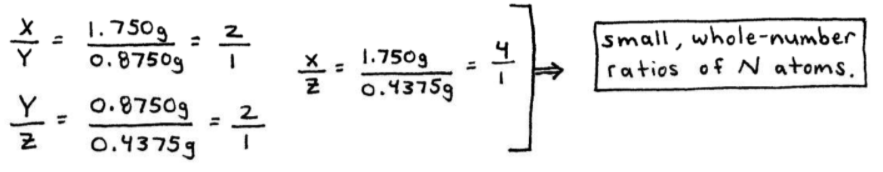# S2E1 - 3 Fundamental Laws of Chemistry, and Dalton's Atomic Theory

Today we are going to discuss the Law of Conservation of Mass, the Law of Definite Proportions, the Law of Multiple Proportions, and Dalton's Atomic Theory.

In General Chemistry there are 3 Fundamental Chemical Laws to be aware of.

## 1. The Law of Conservation of Mass.

Law of Conservation of Mass  =  matter is neither created nor destroyed.

This law is credited to Lavoisier (1743 - 1794).

----------

ex:  Consider the decomposition of water into its elements.

2H2O  →  2H2  +  O2_________

➞  Since matter can neither be created nor destroyed, we must have four hydrogens and two oxygens on both sides of the equation ~~ on both sides of the chemical reaction.

==========

## 2. The Law of Definite Proportions.

Law of Definite Proportions  =  a given compound always contains exactly the same proportion (ratio) of elements by mass.

ex:  water, H2O

contains 2 H's  =  2(1.01)  =  2.02
contains 1 O  =  1(16.00)  =  16.00

➞  the total is the molar mass, or molecular weight of water  =  18.02 g/mol.

Thus, water is always 11.2% H and 88.8% O by mass.In other words, the ratio of H:O atoms in water is always 2H : 1O within the one, definite compound, H2O.

----------

* When John Dalton was on his way to discovering the "atom," he stumbled into, and thus discovered the next law...

==========

## 3. The Law of Multiple Proportions.

Law of Multiple Proportions  =  when 2 elements form a series of compounds, the ratios of the 2nd elements that combine with the first element can be reduced to small whole numbers.

ex:  CO  vs.  CO2

In the multiple compounds above, the ratio of "O" atoms per the one "C" atom is 2:1.

Unlike the Law of Definite Proportions, here we are considering multiple compounds and the ratio is between two of the same type of element:  2"O" : 1"O".

Dalton began to think that because there was always whole-number multiples, there must be some simple repating unit.

This "unit" he called the atom.

For example, one cannot have CO1.5 because you cannot have 0.5 of a "unit" (an oxygen atom).

----------

ex:  Consider 3 compounds that consist of only N and O atoms.

- Compound X consists of 1.750g N and 1g O.

- Compound Y consists of 0.8750g N and 1g O.

- Compound Z consists of 0.4375g N and 1g O.

Show how this data illustrates the Law of Multiple Proportions.
_________

➞  we need to compare the ratio of N atoms by mass in the 3 compounds...==========

## Dalton's Atomic Theory.

In 1808, John Dalton explained the 3 Fundamental Chemical Laws just discussed, with his Atomic Theory.

### Dalton's Postulates

Dalton's Atomic Theory included the following 5 postulates:

1. Each element is composed of tiny indestructible particles called atoms.

2. The atoms of a given element are identical. The atoms of different elements are different.

3. Compounds are formed when atoms of different elements are combined.  A given compound always has the same relative numbers and types of atoms.

4. Chemical reactions involve the re-organization of atoms.  But, the atoms themselves are not changed during the process.

5. Atoms cannot be divided any further.

----------

200 years later, Dalton's Atomic Theory only has two modifications that must be made:

➞  in step 2 above, atoms of a given element are not always identical (isotopes).

➞  in step 5 above, atoms can be divided further into the 3 subatomic particles. Those would be protons, electrons, and neutrons.

==========

Next up in SECTION 2 - Atoms, Molecules, and Ions,

We'll go over those crazy and interesting Early Experiments to Characterize the Atom.